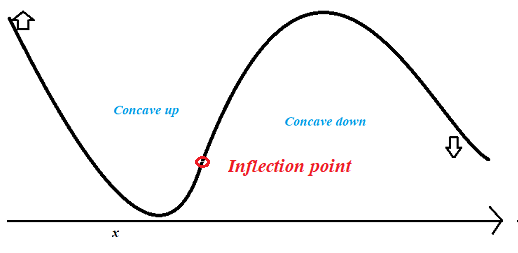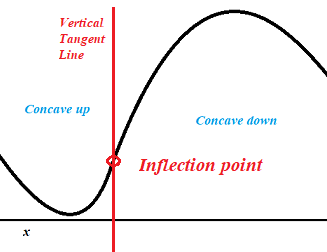# Inflection Point / Turning Point: Definition & Examples

Contents:

## What is an Inflection Point?

An inflection point (sometimes called a turning point, flex , inflection, or “point of diminishing returns”) is where a graph changes curvature, from concave up to concave down or vice versa.

A concave up graph is like the letter U (or, a “cup”), while a concave down graph is shaped like an upside down U, or a Cap (∩). The inflection point happens when a “cup” and a “cap” meet.## Vertical Inflection PointA vertical inflection point, like the one in the above image, has a vertical tangent line; It therefore has an undefined slope and a non-existent derivative.
At first glance, it might not look like there’s a vertical tangent line at the point where the two concavities meet. However, if you zoom in close enough (perhaps with a graphing calculator), you’ll see that there’s a tiny, almost insignificant spot in the graph where the tangent line is perfectly vertical.## Horizontal Points of Inflection

A horizontal point of inflection is where the tangent line is horizontal. The derivative or slope here is zero, because the tangent line is flat.The horizontal inflection point (orange circle) has a horizontal tangent line (orange dashed line).

## Inflection Points and Rates of Change

You can also think of an inflection point as being where the rate of change of the slope changes from increasing to decreasing, or increasing to decreasing.

When slopes of tangent lines increase (from left to right, regardless of sign), your graph is concave up:On the other hand, concave down graphs have decreasing slopes, from left to right:So if you know what the rates of change are at any point on your graph, you can tell where there’s an inflection point.

## Find Inflection Points with The Second Derivatives

The second derivative test uses that information to make assumptions about inflection points. The next graph shows x3 – 3x2 + (x – 2) (red) and the graph of the second derivative of the graph, f” = 6(x – 1) in green. Positive x-values are to the right of the inflection point; Negative x-values are to the left.You can find inflection points by following these general steps :

Step 1: Locate all points where the second derivative equals zero or does not exist. Only include those points where the function is actually defined and there is a tangent line at that point.

Step 2: Create a sign diagram of the second derivative.

Step 3: Choose any point from in Step 1. Determine from the sign diagram in Step 2 whether or not the sign of the second derivative changes as you move across the point. If the sign changes, it is an inflection point.

Example question: Find the inflection points of f(x) = x + (1/x2) .

Step 1: Locate all points where the second derivative equals zero or does not exist. First we need to find the second derivative:Then figure out where the function equals zero or DNE. The function is zero when any of the terms in the numerator equal zero: (6x2 – 2 = 0) or (x2 + 1 = 0).

• Solving (6x2 – 2 = 0) for x, we get x = 1/(√3) or -1/(√3).
• The second equation, (x2 + 1 = 0), has no solutions.

Step 2: Create a sign diagram of the second derivative.Step 3: Determine from the sign diagram in Step 2 whether or not the sign of the second derivative changes as you move across the point. If the sign changes, it is an inflection point. For this example, the sign changes as you move across both points, so x = 1/(√3) and -1/(√3) are both inflection points.

## References

 Sec 4.2 Applications of the Second Derivative. Retrieved July 13, 2021 from: https://mathematics.ku.edu/sites/math.drupal.ku.edu/files/files/Rajaguru_CourseDocuments/class16math115.pdf

CITE THIS AS:
Stephanie Glen. "Inflection Point / Turning Point: Definition & Examples" From StatisticsHowTo.com: Elementary Statistics for the rest of us! https://www.statisticshowto.com/inflection-point/